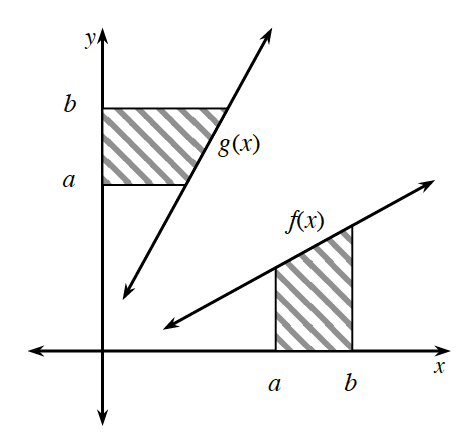### Home > APCALC > Chapter 1 > Lesson 1.3.1 > Problem1-107

1-107.

Sandra is playing around with inverses and thinks she has discovered something interesting. She thinks that if $f(x) = g^{–1}(x)$, then the areas of the regions shaded below are equal. Use $f(x) = \frac { 1 } { 3 }x + 1$ with $a = 3$ and $b = 5$ to verify Sandra’s conjecture.$\text{If }f(x)=\frac{1}{3}x+1\text{ then }f^{-1}(x)=3(x-1)$

$\text{The area of }f(x)=\frac{1}{2}(b-a)\left [ f(b)+f(a) \right ]$

$\text{The area of }g(x)=\frac{1}{2}(b-a)\left [ f^{-1}(a)+f^{-1}(b) \right ]$

Substitute in the values for $a$ and $b$. Are the values equal?